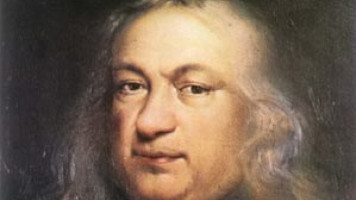Department of Mathematics FAS Harvard University One Oxford Street Cambridge MA 02138 USA Tel: (617) 495-2171 Fax: (617) 495-5132

### Math Table Seminar of October 30, 2019Speaker: Gaurav Goel Title: Fermat's Little Theorem and its Applications to Cryptography Abstract: Fermat's Little Theorem and its cousin, Euler's Totient Theorem, are by far the most applicable results from elementary number theory and hold the key to understanding how most of modern-day public-key cryptography, including the RSA cryptosystem, works. The French mathematician Pierre de Fermat came up with the theorem in the first half of the 17 th century, and it was Leibniz who gave the first documented proof of it in 1683. Leonhard Euler, the famous German mathematician, later introduced his phi function and generalized Fermat's Theorem in 1763 to what is known today as Euler's Totient Theorem. So, what is Fermat's Little Theorem? Why is it called "little"? This talk will explain what Fermat's Little Theorem is and present four proofs of it, including a cool necklace counting argument. It will also discuss briefly Euler's generalization of the theorem. At the end, I will present the applications of the developed theory to cryptography, including an explanation of the Pohling-Hellman exponential cryptosystem and finally the RSA cryptosystem. As usual dinner will be provided.